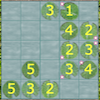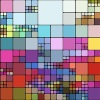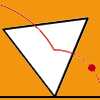# Resources tagged with: Interactivities

Filter by: Content type:
Age range:
Challenge level:

### There are 94 results

Broad Topics > Physical and Digital Manipulatives > Interactivities### Triangles in Circles

##### Age 11 to 14Challenge Level

Can you find triangles on a 9-point circle? Can you work out their angles?### Right Angles

##### Age 11 to 14Challenge Level

Can you make a right-angled triangle on this peg-board by joining up three points round the edge?### Subtended Angles

##### Age 11 to 14Challenge Level

What is the relationship between the angle at the centre and the angles at the circumference, for angles which stand on the same arc? Can you prove it?### Semi-regular Tessellations

##### Age 11 to 16Challenge Level

Semi-regular tessellations combine two or more different regular polygons to fill the plane. Can you find all the semi-regular tessellations?### Bow Tie

##### Age 11 to 14Challenge Level

Show how this pentagonal tile can be used to tile the plane and describe the transformations which map this pentagon to its images in the tiling.### Matter of Scale

##### Age 14 to 16Challenge Level

Prove Pythagoras' Theorem using enlargements and scale factors.### Estimating Angles

##### Age 7 to 14Challenge Level

How good are you at estimating angles?### Tessellation Interactivity

##### Age 7 to 16Challenge Level

An environment that enables you to investigate tessellations of regular polygons### Rolling Around

##### Age 11 to 14Challenge Level

A circle rolls around the outside edge of a square so that its circumference always touches the edge of the square. Can you describe the locus of the centre of the circle?### Colour in the Square

##### Age 7 to 16Challenge Level

Can you put the 25 coloured tiles into the 5 x 5 square so that no column, no row and no diagonal line have tiles of the same colour in them?### Overlap

##### Age 11 to 14Challenge Level

A red square and a blue square overlap so that the corner of the red square rests on the centre of the blue square. Show that, whatever the orientation of the red square, it covers a quarter of the. . . .### Tilting Triangles

##### Age 14 to 16Challenge Level

A right-angled isosceles triangle is rotated about the centre point of a square. What can you say about the area of the part of the square covered by the triangle as it rotates?### Spinners Environment

##### Age 5 to 18Challenge Level

A tool for generating random integers.### Nine Colours

##### Age 11 to 16Challenge Level

Can you use small coloured cubes to make a 3 by 3 by 3 cube so that each face of the bigger cube contains one of each colour?##### Age 14 to 16Challenge Level

A counter is placed in the bottom right hand corner of a grid. You toss a coin and move the star according to the following rules: ... What is the probability that you end up in the top left-hand. . . .### Inside Out

##### Age 14 to 16Challenge Level

There are 27 small cubes in a 3 x 3 x 3 cube, 54 faces being visible at any one time. Is it possible to reorganise these cubes so that by dipping the large cube into a pot of paint three times you. . . .### Latin Lilies

##### Age 7 to 14Challenge Level

In this game you are challenged to gain more columns of lily pads than your opponent.### Excel Interactive Resource: Interactive Division

##### Age 11 to 16Challenge Level

Use an Excel to investigate division. Explore the relationships between the process elements using an interactive spreadsheet.### A Tilted Square

##### Age 14 to 16Challenge Level

The opposite vertices of a square have coordinates (a,b) and (c,d). What are the coordinates of the other vertices?### Factors and Multiples Game

##### Age 7 to 16Challenge Level

A game in which players take it in turns to choose a number. Can you block your opponent?### Poly Plug Rectangles

##### Age 5 to 14Challenge Level

The computer has made a rectangle and will tell you the number of spots it uses in total. Can you find out where the rectangle is?### Attractive Tablecloths

##### Age 14 to 16Challenge Level

Charlie likes tablecloths that use as many colours as possible, but insists that his tablecloths have some symmetry. Can you work out how many colours he needs for different tablecloth designs?### Squaring the Circle and Circling the Square

##### Age 14 to 16Challenge Level

If you continue the pattern, can you predict what each of the following areas will be? Try to explain your prediction.### Disappearing Square

##### Age 11 to 14Challenge Level

Do you know how to find the area of a triangle? You can count the squares. What happens if we turn the triangle on end? Press the button and see. Try counting the number of units in the triangle now. . . .### Interactive Number Patterns

##### Age 14 to 16Challenge Level

How good are you at finding the formula for a number pattern ?### Got It

##### Age 7 to 14Challenge Level

A game for two people, or play online. Given a target number, say 23, and a range of numbers to choose from, say 1-4, players take it in turns to add to the running total to hit their target.### Number Pyramids

##### Age 11 to 14Challenge Level

Try entering different sets of numbers in the number pyramids. How does the total at the top change?### Picturing Triangular Numbers

##### Age 11 to 14Challenge Level

Triangular numbers can be represented by a triangular array of squares. What do you notice about the sum of identical triangle numbers?### Icosian Game

##### Age 11 to 14Challenge Level

This problem is about investigating whether it is possible to start at one vertex of a platonic solid and visit every other vertex once only returning to the vertex you started at.### Last Biscuit

##### Age 11 to 18Challenge Level

Can you find a strategy that ensures you get to take the last biscuit in this game?### Proof Sorter - Quadratic Equation

##### Age 14 to 18Challenge Level

This is an interactivity in which you have to sort the steps in the completion of the square into the correct order to prove the formula for the solutions of quadratic equations.### Square It

##### Age 11 to 16Challenge Level

Players take it in turns to choose a dot on the grid. The winner is the first to have four dots that can be joined to form a square.### Sliding Puzzle

##### Age 11 to 16Challenge Level

The aim of the game is to slide the green square from the top right hand corner to the bottom left hand corner in the least number of moves.### Cogs

##### Age 11 to 14Challenge Level

A and B are two interlocking cogwheels having p teeth and q teeth respectively. One tooth on B is painted red. Find the values of p and q for which the red tooth on B contacts every gap on the. . . .### More Number Pyramids

##### Age 11 to 14Challenge Level

When number pyramids have a sequence on the bottom layer, some interesting patterns emerge...### Rollin' Rollin' Rollin'

##### Age 11 to 14Challenge Level

Two circles of equal radius touch at P. One circle is fixed whilst the other moves, rolling without slipping, all the way round. How many times does the moving coin revolve before returning to P?### Guesswork

##### Age 14 to 16Challenge Level

Ask a friend to choose a number between 1 and 63. By identifying which of the six cards contains the number they are thinking of it is easy to tell them what the number is.### Partitioning Revisited

##### Age 11 to 14Challenge Level

We can show that (x + 1)² = x² + 2x + 1 by considering the area of an (x + 1) by (x + 1) square. Show in a similar way that (x + 2)² = x² + 4x + 4### Tilted Squares

##### Age 11 to 14Challenge Level

It's easy to work out the areas of most squares that we meet, but what if they were tilted?### Changing Places

##### Age 14 to 16Challenge Level

Place a red counter in the top left corner of a 4x4 array, which is covered by 14 other smaller counters, leaving a gap in the bottom right hand corner (HOME). What is the smallest number of moves. . . .### Jam

##### Age 14 to 16Challenge Level

To avoid losing think of another very well known game where the patterns of play are similar.### Excel Interactive Resource: Fraction Multiplication

##### Age 11 to 16Challenge Level

Use Excel to explore multiplication of fractions.### Squaring the Rectangle

##### Age 14 to 18Challenge Level

Can you find a way to turn a rectangle into a square?### Speeding Up, Slowing Down

##### Age 11 to 14Challenge Level

Experiment with the interactivity of "rolling" regular polygons, and explore how the different positions of the red dot affects its speed at each stage.### Just Rolling Round

##### Age 14 to 16Challenge Level

P is a point on the circumference of a circle radius r which rolls, without slipping, inside a circle of radius 2r. What is the locus of P?### Excel Interactive Resource: Haitch

##### Age 11 to 16Challenge Level

An Excel spreadsheet with an investigation.### Excel Interactive Resource: Fraction Addition & Fraction Subtraction

##### Age 11 to 16Challenge Level

Use Excel to practise adding and subtracting fractions.### Excel Interactive Resource: Multiples Chain

##### Age 11 to 16Challenge Level

Use an interactive Excel spreadsheet to investigate factors and multiples.### Excel Interactive Resource: Long Multiplication

##### Age 11 to 16Challenge Level

Use an Excel spreadsheet to explore long multiplication.### Magic W

##### Age 14 to 16Challenge Level

Find all the ways of placing the numbers 1 to 9 on a W shape, with 3 numbers on each leg, so that each set of 3 numbers has the same total.